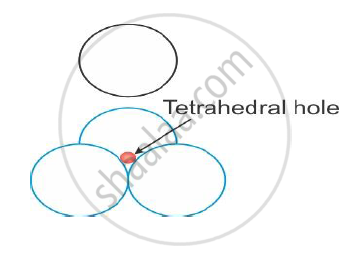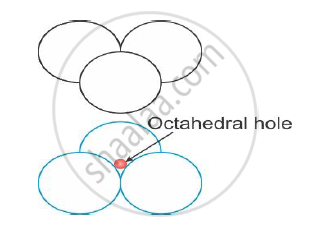Department of Pre-University Education, KarnatakaPUC Karnataka Science Class 12

# How Will You Distinguish Between the Following Pairs of Terms: Tetrahedral and Octahedral Voids - Chemistry

Distinguish Between

How will you distinguish between the following pairs of terms: Tetrahedral and octahedral voids

#### Solution

Tetrahedral voids and octahedral voids:

 Tetrahedral voids Octahedral voids The tetrahedral void is the name given to the vacant space formed by touching of four spheres, as shown in the figure.Octahedral voids are formed by combination of voids of two triangular layers of atoms, when one triangular layer is placed above another, as shown in the figure,Concept: Close Packed Structures - Formula of a Compound and Number of Voids Filled
Is there an error in this question or solution?